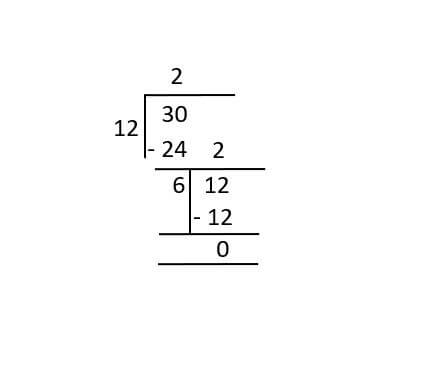# Greatest Common Factor (GCF)

## Find the highest common factor of the given numbers

Home

Math

Greatest Common Factor

You can input in any of the fields and get equivalent values.
=
=

## What is GCF?• Greatest Common Factor

### A factor is the number which divides another number without leaving a remainder. for eg: 2 , 3 , 4 , 6 are the factors of 12.

• What Methods are there to find GCF (GCD/HCF)?

## Division Method to find GCF## Examples: GCF of 12 and 30 using Division method.

• ### Division Flow## Prime Factorization method## Grid Method

• ### Step 3: Continue dividing the numbers till the last common factor.Product of the prime numbers in the left side grid will be the gcf of the numbers.## Table of GCF / HCF of Most Common NumbersGCF Input

HCF Input

### Frequently Asked Questions on GCF.